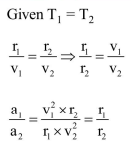# Two cars of masses

Question:

Two cars of masses $m_{1}$ and $m_{2}$ are moving in circles of radii $r_{1}$ and $r_{2}$, respectively. Their speeds are such that they make complete circles in the same time t. The ratio of their centripetal acceleration is :-

1. $1: 1$

2. $\mathrm{m}_{1} \mathrm{r}_{1}: \mathrm{m}_{2} \mathrm{r}_{2}$

3. $\mathrm{m}_{1}: \mathrm{m}_{2}$

4. $r_{1}: r_{2}$

Correct Option: , 4

Solution: